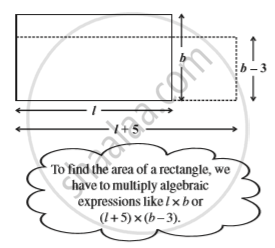Share

# Multiplication of Algebraic Expressions: Introduction

#### notes

For instance , Ameena gets up. She says, “We can think of area of a rectangle.” The area of a rectangle is l × b, where l is the length, and b is breadth. If the length of the rectangle is increased by 5 units, i.e., (l + 5) and breadth is decreased by 3 units , i.e., (b – 3) units, the area of the new rectangle will be (l + 5) × (b – 3).### Shaalaa.com

Multiplication of Algebraic Expressions: Introduction [00:05:59]
S
0%

S